# Math in Focus Grade 2 Chapter 6 Answer Key Multiplication Tables of 2, 5, and 10

Go through the Math in Focus Grade 2 Workbook Answer Key Chapter 6 Multiplication Tables of 2, 5, and 10 to finish your assignments.

## Math in Focus Grade 2 Chapter 6 Answer Key Multiplication Tables of 2, 5, and 10

Math Journal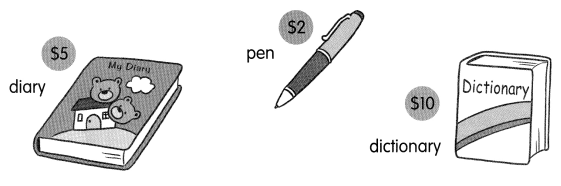Look at the pictures.
Write multiplication stories.
Use multiplication tables of 5 and 10.

Example
Mandy bought 10 pens.
She paid $2 for each pen. How much did she pay altogether? Story A Sunny bought two diary’s He paid$ 5 for each diary
Multiply 5 with 2 we get the total cost
2 × 5 = 10
He paid $10 in total Look at the pictures on page 149. Write multiplication stories. Use multiplication tables of 5 and 10 Story B Joe bought 6 dictionaries He paid$10 for each dictionaries
Multiply 10 with 6 we get the total cost
10 × 6 = 60
He paid $60 in total Story C Mario bought 5 pens He paid$2 for each pen
Multiply 5 with 2 we get the total cost
5 × 2 = 10
He paid \$10 in tota

Challenging Practice

Fill in the blanks with the correct numbers.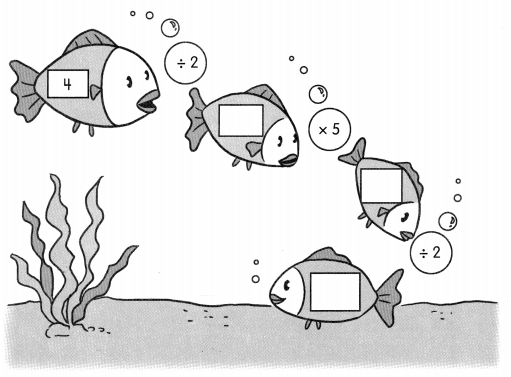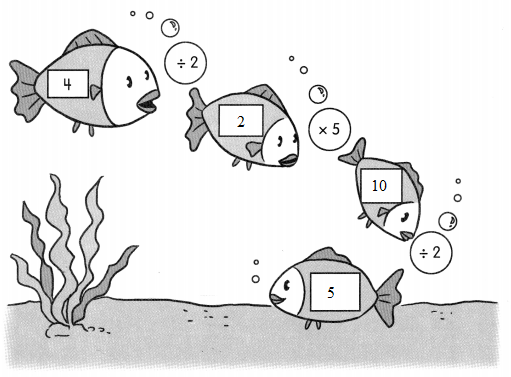Problem Solving

Solve.

A shop sells oranges and apples packed as shown.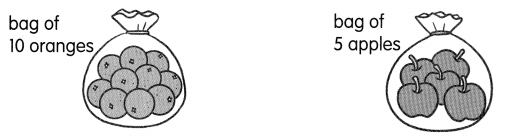For every 10 oranges Jennifer buys, she also buys 5 apples. She buys 20 oranges. How many bags of fruits does she buy altogether?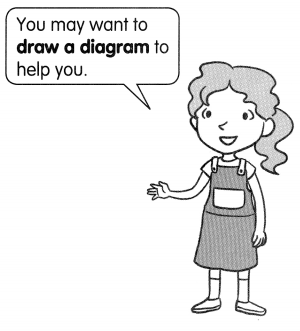Jennifer buys ____ bags of fruits altogether.
Jennifer buys 4 bags of fruits altogether.
explanation:
Given:
One bag of oranges contain = 10 oranges
One bag of apple contain = 5 apples
For every 10 oranges she buys 5 apples
so 2 bags of oranges and 2 bags of apples
Total of 4 bags

Chapter Review/Test

Vocabulary

Fill in the blanks.

Circle the words that belong.

Question 1.
15, ___, 25, 30, ___, 40
To find the answer, (multiply / skip-count) by fives.
15,20,25,30,35,40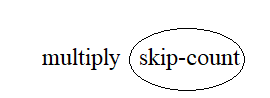Question 2.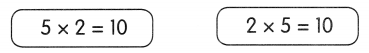These are (addition facts / related multiplication facts).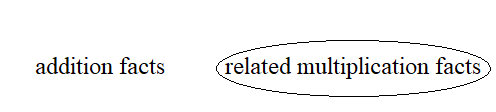Concepts and Skills

Use dot paper to find the missing numbers.

Question 3.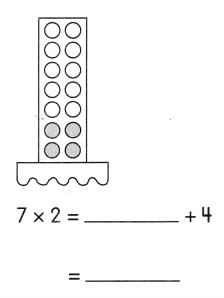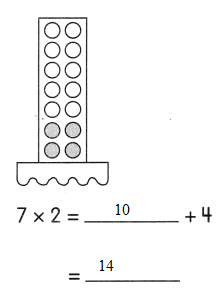Question 4.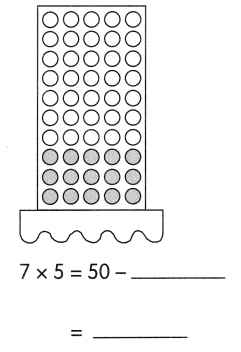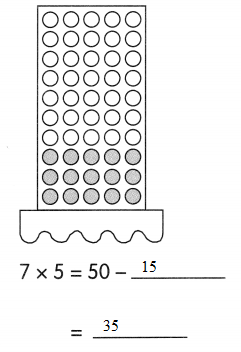Use dot paper to find the missing numbers.

Question 5.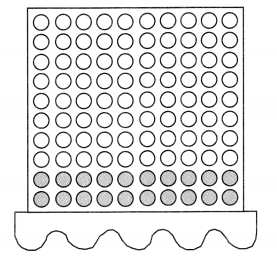8 × 10 = 100 – ___
= ____________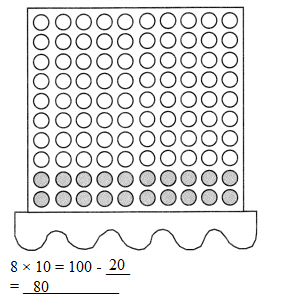Question 6.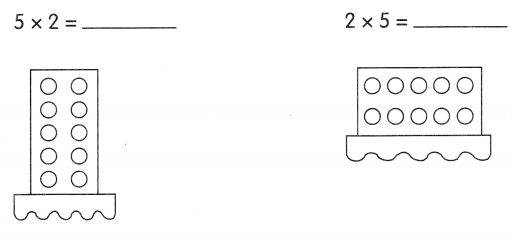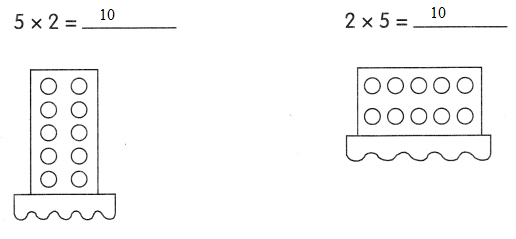Question 7.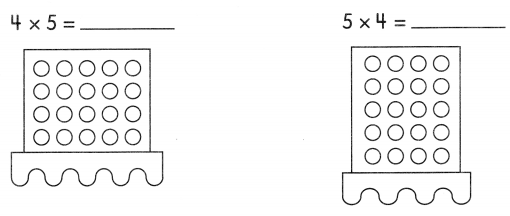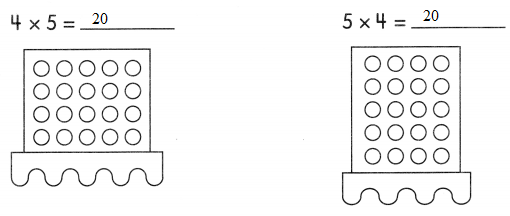Use dot paper to find the missing numbers.

Question 8.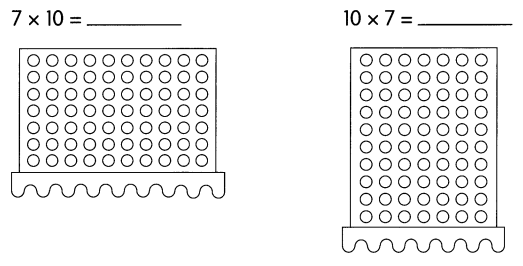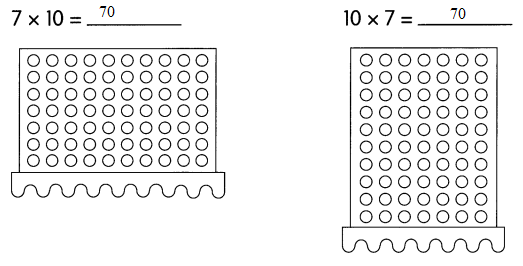Find the missing numbers. Then match the numbers to the letters to answer the riddle.

Question 9.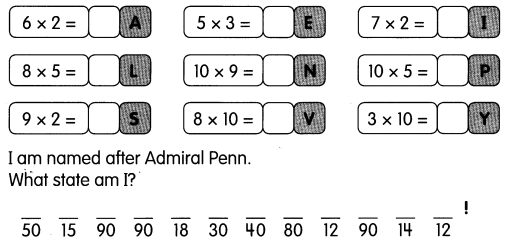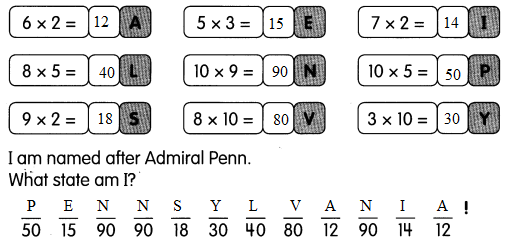Use related multiplication facts to find the missing numbers.

Question 10.
4 × 2 = 8
So, 8 ÷ 2 = ___
4 × 2 = 8
So, 8 ÷ 2 = 4

Question 11.
3 × 5 = 8
So, 15 ÷ 3 = ___
3 × 5 = 8
So, 15 ÷ 3 = 5

Question 12.
9 × 10 = 90
So, 90 ÷ 10 = ___
9 × 10 = 90
So, 90 ÷ 10 = 9

Question 13.
5 × 10 = 50
So, 50 ÷ 5 = ___
5 × 10 = 50
So, 50 ÷ 5 = 10

Problem Solving

Use  to solve.

Question 14.
Teddy grills 8 skewers of chicken. Each skewer has 2 pieces of chicken. How many pieces of chicken does he grill?
He grills ___ pieces of chicken.
Explanation:
Given:
Teddy grills skewers of chicken = 8
Each skewer has = 2 pieces
by using skip counting method we get the total pieces of chicken
8 × 2 = 16 pieces

Question 15.
Naomi pastes 9 star stickers on each piece of paper. There are 5 pieces of paper. How many star stickers are on the pieces of paper? There are ____ star stickers on the pieces of paper.
Explanation:
Given:
Total star stickers pasted on each piece of paper = 9
Total number of pieces of paper = 5 pieces
by using skip counting method we get the total pieces of chicken
9 × 5 = 45 pieces

Use related multiplication facts to solve.

Question 16.
Mr. Wilson picks 60 strawberries. He gives an equal number of strawberries to 10 children. How many strawberries does each child get?
Each child gets ___ strawberries.
Explanation:
Given:
Total number of strawberries Mr. Wilson picks = 60
Total number of children present = 10
60 ÷ 10  = 6
So, each child get 6 strawberries
as 10 × 6 = 60

Question 17.
Jess gives away 20 pennies. She gives each friend 5 pennies. How many friends are there? There are ___ friends.